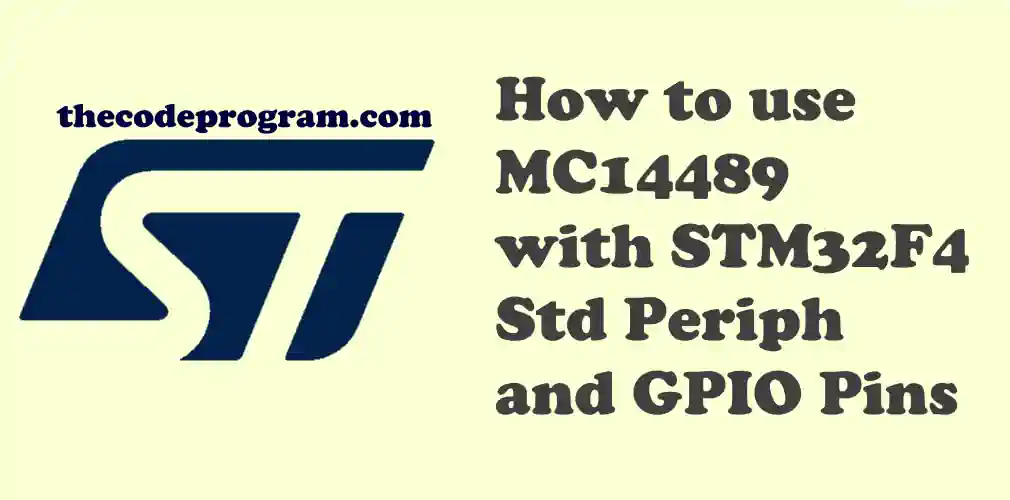## How to use MC14489 with STM32F4 Std Periph and GPIO Pins

Hello everyone, in this article we are going to talk about usage of MC14489 with STM32F4 STD Periph Libraries and GPIO layers. We will use soft SPI protocol drive 5x7 Segment Display.

Let's Get Started.

This application is the same application at How to use MC14489 with Arduino Counter Example

Firstly, What is MC14489
MC14489 is a LED driver which can be interfaced directly diodes. We can drive 7 segment displays or variations of this. LED's must be connected as common cathode logic. We can control maximum 5 digits with one MC14489 micro controller. MC14489 can be interfaced with SPI communication protocol. It accepts 24-bit data. We send all 24 digits numbers in 24bit . We can also send the configuration data with 8-bits.

Important Note : I always recommend to read the datasheet firstly.

In this application I have connected the below pins:
• PB15 Data In
• PB13 Clock

Now let's start coding.

Firstly we need to declare below variable for our programs.
``````
uint32_t delay_counter;
unsigned char digits;
``````

Now we need to write our essential method. This method will send the data to MC14489 over Soft SPI method. Below code block will open/close the clock pin and during this operation it will set the data pin according to data bit.

``````
void write_MC14489( uint8_t digitData) {

int i=0;
int pinState;

GPIO_ResetBits(GPIOB, GPIO_Pin_13);
GPIO_ResetBits(GPIOB, GPIO_Pin_15);

for (i=7; i>=0; i--)  {
GPIO_ResetBits(GPIOB, GPIO_Pin_13);
if ( digitData & (1<<i) ) {
GPIO_SetBits(GPIOB, GPIO_Pin_15);
}
else {
GPIO_ResetBits(GPIOB, GPIO_Pin_15);
}

GPIO_SetBits(GPIOB, GPIO_Pin_13);
GPIO_ResetBits(GPIOB, GPIO_Pin_15);
}

GPIO_ResetBits(GPIOB, GPIO_Pin_13);
}
``````
Now we need to write digits to 7 Segment Display. Below code block will call above code and it will send the digits to MC14489 bit by bit.
``````
void writeDigits(unsigned char digits)
{
GPIO_ResetBits(GPIOE, GPIO_Pin_7);

write_MC14489(  (0xff << 4) | digits  ); // D23~D16
write_MC14489(  (digits << 4) | digits); // D15~D8
write_MC14489(  (digits << 4) | digits ); // D7~D0

GPIO_SetBits(GPIOE, GPIO_Pin_7);
}
``````
We will first start the display. Calling below method will start the display.
``````
void startDisplay()
{
GPIO_ResetBits(GPIOE, GPIO_Pin_7);

write_MC14489( 0x01);

GPIO_SetBits(GPIOE, GPIO_Pin_7);
}
``````
Below code block will start the second counter of the program.
``````
void startCounter(){

for(int i=0; i<100000; i++){
digits = i % 100000 /10000;
digits = i % 10000  /1000;
digits = i % 1000   /100;
digits = i % 100   / 10 ;
digits = i % 10     ;

writeDigits(digits);

delay_counter = 8000000;
while(delay_counter--);
}
}
``````
Our functions are ready now. Below main function will enable the GPIO's and then start the display first and then start the counter.
``````
int main()
{
RCC_AHB1PeriphClockCmd(RCC_AHB1Periph_GPIOB, ENABLE);
RCC_AHB1PeriphClockCmd(RCC_AHB1Periph_GPIOE, ENABLE);

GPIO_InitTypeDef GPIO_Structure;

GPIO_Structure.GPIO_Mode = GPIO_Mode_OUT;
GPIO_Structure.GPIO_Pin = GPIO_Pin_13 | GPIO_Pin_15;
GPIO_Structure.GPIO_OType = GPIO_OType_PP;
GPIO_Structure.GPIO_Speed = GPIO_Speed_25MHz;
GPIO_Init(GPIOB, &GPIO_Structure);

GPIO_Structure.GPIO_Mode = GPIO_Mode_OUT;
GPIO_Structure.GPIO_Pin =  GPIO_Pin_7 | GPIO_Pin_8;
GPIO_Structure.GPIO_OType = GPIO_OType_PP;
GPIO_Structure.GPIO_PuPd = GPIO_PuPd_UP;
GPIO_Structure.GPIO_Speed = GPIO_Speed_25MHz;
GPIO_Init(GPIOE, &GPIO_Structure);

startDisplay();

while(1)
{
startCounter();

delay_counter = 65000;
while(delay_counter--);
}
}
``````

Burak Hamdi TUFAN

12/04/2020

01/11/2020

14/02/2021